Definitions of Square Dance Calls and Concepts
Stable Concept [C3A]Index -->  Plus  |  A1  |  A2  |  C1  |  C2  |  C3A  |  C3B  |  C4  |  NOL  |
Definitions (Text Only) -->  Plus  |  A1  |  A2  |  C1  |  C2  |  C3A  |  C3B  |  C4  |  NOL  |
 Find call:

 \$B8@8l(BDo the given call without changing your facing direction. Your ending position will be the same as if you had executed the call normally, but your facing direction will not change from your original facing direction. EN: 10
\$BM?\$(\$i\$l\$?%3!<%k\$r8~\$-\$rJQ\$(\$:\$K9T\$\$\$^\$9!%=*\$o\$j\$N0LCV\$O%3!<%k\$rIaDL\$K9T\$C\$?\$H\$-\$HF1\$8\$G\$9\$,(B, \$B8~\$-\$O%3!<%k\$r;O\$a\$k\$H\$-\$HJQ\$o\$j\$^\$;\$s!%(B JP: 10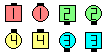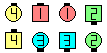Stable In Roll Circulate\$B\$NA0(B \$B8e(B

Note: for most calls, try to dance the entire call without changing your facing direction. For more complicated calls (such as Stable Scoot Reaction), if you feel you are unable to dance the entire call Stable, you can cheat by memorizing the wall that you are facing, doing the call, and then turning to face that wall. EN: 20
\$BCmStable \$B\$G\$O\$G\$-\$J\$\$\$H;W\$C\$?\$H\$-(B, \$B8~\$\$\$F\$\$\$kJI\$r3P\$(\$F\$*\$\$\$F%3!<%k\$r9T\$\$(B, \$B3P\$(\$F\$*\$\$\$?JI\$X8~\$-\$rJQ\$(\$k\$H\$\$\$&(B cheat \$B\$,;H\$(\$^\$9!%(B JP: 20

\$B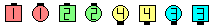\$B\$B;O\$a\$N(B formation: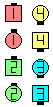Stable As Couples Bend The Line\$B\$N8e(B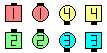As Couples Stable Bend The Line\$B\$N8e(B

1/4 Stable CONCEPT [C4] (Vic Ceder 1983):
Start the given call normally but after turning 90° do the remainder of the call Stable. EN: 209
90°\$B2s\$k\$^\$G\$OM?\$(\$i\$l\$?%3!<%k\$rIaDL\$K9T\$\$(B, \$B\$=\$N8e(B, \$B%3!<%k\$N;D\$j\$r(B Stable \$B\$G9T\$\$\$^\$9!%(B JP: 209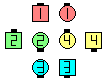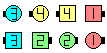1/4 Stable Little More\$B\$NA0(B \$B8e(B

n/4 Stable CONCEPT [C4] (Vic Ceder 1983):
Start the given call normally but after turning a cumulative total of n · 90° do the remainder of the call Stable. Note that the turning is cumulative: if you turn 90° right and then 90° left, it is considered that you have turned a total of 180° (not 0°). Ponder the difference between a 3/4 Stable Exchange The Diamond and a Stable 3/4 Exchange The Diamond. EN: 210
\$BN_@QE*\$K(B n · 90°\$B2s\$k\$^\$G\$OM?\$(\$i\$l\$?%3!<%k\$rIaDL\$K9T\$\$(B, \$B\$=\$N8e(B, \$B%3!<%k\$N;D\$j\$r(B Stable \$B\$G9T\$\$\$^\$9!%2sE>\$,N_@QE*\$H\$\$\$&\$3\$H\$O(B, \$BNc\$(\$P(B 90°\$B1&\$X2s\$j\$=\$7\$F(B 90°\$B:8\$X2s\$k\$H\$\$\$&\$3\$H\$O(B, \$B9g7W\$G(B 180°(0°\$B\$G\$J\$/(B) \$B2s\$k\$3\$H\$K\$J\$j\$^\$9!%(B3/4 Stable Exchange The Diamond \$B\$H(B Stable 3/4 Exchange The Diamond \$B\$N0c\$\$\$rNI\$/9M\$(\$F\$_\$F\$/\$@\$5\$\$!%(B JP: 210CALLERLAB definition for Stable ConceptChoreography for Stable ConceptComments? Questions? Suggestions?